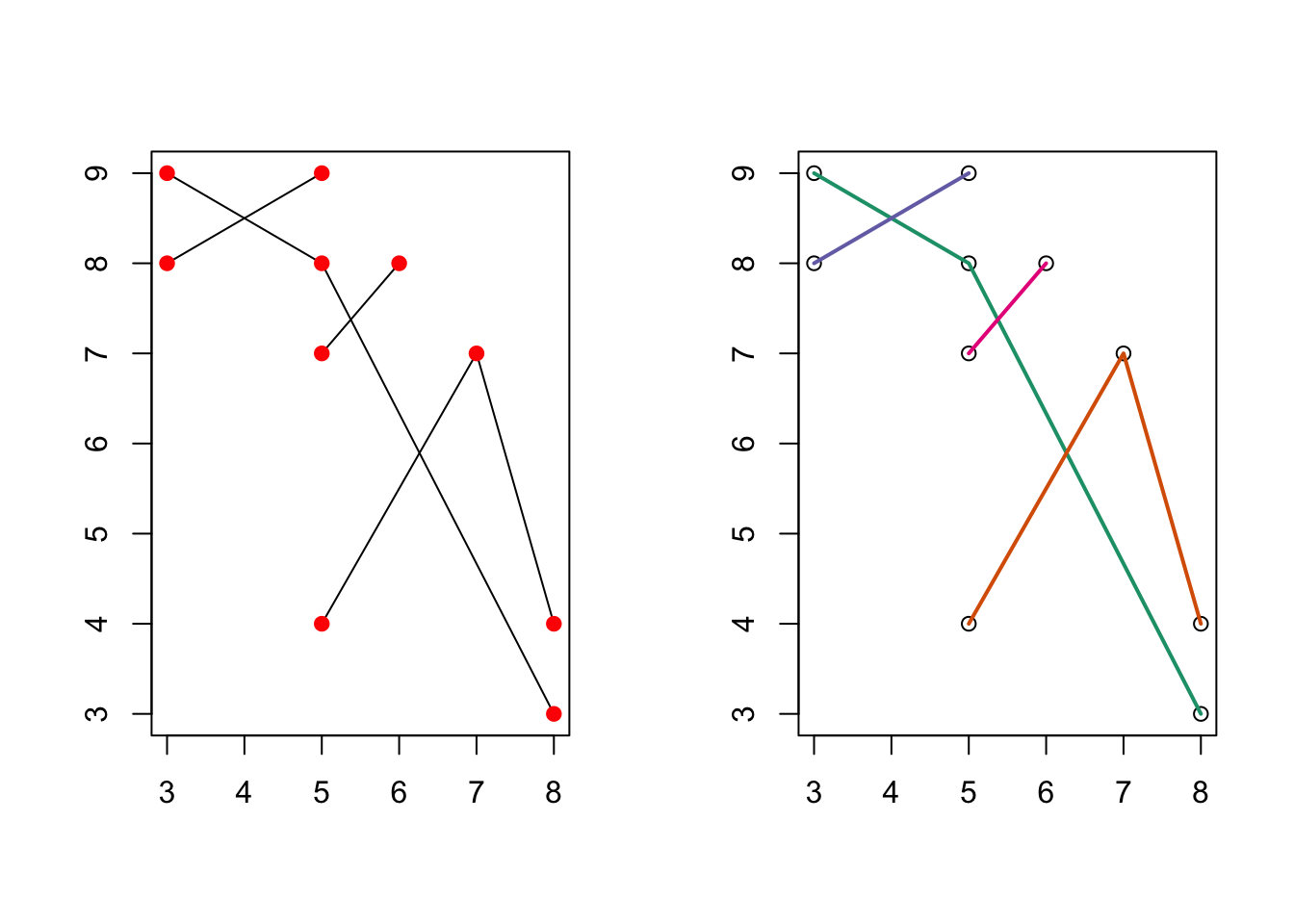Contact : Arthur Charpentier charpentier.arthur@uqam.ca

rm(list=ls())

We will use some some network packages (mainly to illustrate)

library(igraph)
library(shp2graph)

as well as some packages for spatial analysis, and visualization

library(geosphere)
library(rgdal)
library(leaflet)
library(scales)
library(lwgeom)
library(shapefiles)
library(sp)
library(rgeos)
library(osmdata)
library(sf)
library(ggmap)
library(maptools)

For technical reasons, we need additional packages

library(Matrix)
library(tripack)
library(RColorBrewer)
library(units)
library(pals)
library(classInt)

In the applications, we will use gurobi. It is a commercial optimization solver for linear programming, quadratic programming (among many other), that is available for free in academia. Installation can be a little bit complicated (see the vignette online),

library('gurobi')

We will consider three applications in this guide

• the toy example just to explain the four components in our approach : the nodes and the edges (a road network for instance), the location of sellers and buyers. The first part is to match locations of sellers and buyers with nodes. Then we solve the linear programming problem.
• the metro example to illustrate the use of a real network (here the metro in Paris), with a single buyer and several sellers (hospitals here)
• the maternity example to illustrate on read data, with all maternities in France (sellers) and all pregnant women (buyers).

# 1 The Theoretical Model

The geometry of the geographic market is described by a connected graph $$G=\left(V,E\right)$$ where $$V$$ is a set of vertices and $$E$$ is a set of directed edges. Vertices are the different locations; edges are road segments linking two adjacent locations.

Agents are located at the vertices of our graph (if not, as we will see, we will match them with the closest vertice).We make the following assumptions on their consumption preferences:

• (A1) Consumers with the same location have the same outside option,
• (A2) Consumers have the same cost of travel along a given edge,
• (A3) There is no capacity constraints on the supply side.

A path $$\pi_{vw}$$ between two nodes $$v,w\in V$$ is a sequence of connected edges starting in $$v$$ and ending in $$w$$. We note $$\Pi_{vw}$$ the set of paths between $$v$$ and $$w$$.

For each edge $$e\in E$$, we define $$c_{e}$$ the cost of travel along this arc. A seller located in $$w\in V$$ sells at an endogenous price $$p_{w}$$. Therefore a consumer located in $$v$$ chooses to buy from the seller located in $$w$$ that minimizes his total cost $$p_{v}$$ $p_{v}=\underset{w\in V\text{, }\pi_{vw}\in\Pi_{vw}}{\min}\left[p_{w}+\underset{e\in\pi_{vw}}{\sum}c_{e}\right]$

Let’s denote$$u_{v}$$ the reservation utility of agents located in $$v$$ and $$p_{v}$$ the price for these agents (including the cost of transportation). Therefore, if $$p_{v}>u_{v}$$ a consumer in $$v$$ his outside option, else he buys from the most competitive seller depending on his location in the market.

This outside option can be modeled by a fictitious node $$0$$, where the price of the commodity is set to $$p_{0}=0$$ and the cost of travel is $$c_{v0}=u_{v}$$.

Hence the excess demand $$z_{v}$$ at a node $$v\in V$$ does not depend on the price. We are solving a matching problem over a network between sellers and consumers.

At equilibrium each consumer chooses the most competitive seller depending on his location; furthermore the demand received by each seller must equal his supply. If we define $$\mu_{e}$$ the number of agents traveling along edge $$e$$, these equilibrium conditions can be written: $\left\{ \begin{array}{l} \forall vw\in E\text{, }p_{w}-p_{v}\leq c_{vw}\\ \forall vw\in E\text{ such that }\mu_{vw}>0\text{, }p_{w}-p_{v}=c_{vw}\\ \forall v\in V\text{, }\underset{w\in V}{\sum}\mu_{vw}-\underset{u\in V}{\sum}\mu_{uv}=z_{v} \end{array}\right.$

These equations are the complementary slackness conditions for a minimum cost flow problem over network $$G$$. Hence, if the graph is connected and if there isn’t any loop of negative cost in $$G$$, there exists an equilibrium which is the solution of the min-cost flow problem $\begin{array}{cl} \mu=\text{argmin}\underset{e\in E}{\sum}c_{e}\mu_{e}\\ & \mu\text{ s.t. }\forall v\in V\text{, }\underset{w\in V}{\sum}\mu_{vw}-\underset{u\in V}{\sum}\mu_{uv}=z_{v} \end{array}$

Models used in trade theory usually use iceberg transport costs. These costs are linear in distance and paid in the transported good once arrived - hence the metaphor of the iceberg that melts as you transport it.

Our model can incorporate these costs applying a log-transformation to the equilibrium conditions. Let’s define for all $$vw\in E$$ iceberg costs $$\tau_{vw}$$. An equilibrium $$\left(\mu,p\right)$$ should satisfy $\left\{ \begin{array}{l} \forall vw\in E\text{, }\left(1-\tau_{vw}\right)p_{w}\leq p_{v}\\ \forall vw\in E\text{ such that }\mu_{vw}>0\text{, }\left(1-\tau_{vw}\right)p_{w}=p_{v}\\ \forall v\in V\text{, }\underset{w\in V}{\sum}\mu_{vw}-\underset{u\in V}{\sum}\mu_{uv}=z_{v} \end{array}\right.$ which becomes $\left\{ \begin{array}{l} \forall vw\in E\text{, }\log p_{w}-\log p_{v}\leq-\log\left(1-\tau_{vw}\right)\\ \forall vw\in E\text{ such that }\mu_{vw}>0\text{, }\log p_{w}-\log p_{v}=-\log\left(1-\tau_{vw}\right)\\ \forall v\in V\text{, }\underset{w\in V}{\sum}\mu_{vw}-\underset{u\in V}{\sum}\mu_{uv}=z_{v} \end{array}\right.$

Therefore this problem is equivalent to the one presented earlier with $$\forall vw\in E$$, $$c_{vw}=-\log\left(1-\tau_{vw}\right)$$, and replacing $$p$$ by its logarithm.

# 2 A Toy Example

## 2.1 Networks and Shapefiles

Consider a shapefile of roads, or train lines : each road is a collection of segments, or connected nodes. Our shapefile is, so fact, only in a dataframe dd

dd <- data.frame(Id=c(1,1,1,2,2,2,3,3,4,4),
X=c(3,5,8,5,7,8,3,5,5,6),
Y=c(9,8,3,4,7,4,8,9,7,8))

Here are the (given) knots and the four roads

par(mfrow=c(1,2))
plot(dd$X,dd$Y,col="white",xlab="",ylab="")
for(i in unique(dd[,1])) lines(dd$X[dd$Id==i],dd$Y[dd$Id==i])
points(dd$X,dd$Y,pch=19,col="red")
library(RColorBrewer)
darkcols = brewer.pal(8, "Dark2")
plot(dd$X,dd$Y,xlab="",ylab="")
for(i in unique(dd[,"Id"])) lines(dd$X[dd$Id==i],dd$Y[dd$Id==i],col=darkcols[i],lwd=2)Let us create a shapefile from those segments

Show code for the extend_shp() function
dd2 <- extend_shp(dd)

par(mfrow=c(1,2))
plot(dd$X,dd$Y,col="white",xlab="",ylab="")
for(i in unique(dd[,1])) lines(dd$X[dd$Id==i],dd$Y[dd$Id==i])
points(dd2[["knots"]]$X,dd2[["knots"]]$Y,pch=19,col="blue")
points(dd$X,dd$Y,pch=19,col="red")
plot(dd2[["shp"]]$X,dd2[["shp"]]$Y,col="white",xlab="",ylab="",axes=FALSE,xlim=c(2.6,8.4))
text(dd2[["knots"]]$X,dd2[["knots"]]$Y,dd2[["knots"]]$NO) points(dd2[["knots"]]$X,dd2[["knots"]]\$Y,cex=3)